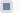Victor L. Mironov OctonsSite menuInput formSearchStatistics Total online: 1 Guests: 1 Users: 0Welcome, Guest · RSS 26.11.2020, 07:23

# Algebra of octons

The eight-component octon Ğ is defined in the following form

Ğ=d + a+ b+ c+ D+ A+ B+ CK

where values i, jare polar unit vectors; IJK are axial unit vectors and Е is the pseudoscalar unit. The octon’s components d, a, b, c, D, A, B, C are the numbers (complex in general). Thus the octon is the sum of scalar, vector, pseudoscalar and pseudovector and the i, jk, IJvalues are polar and axial bases of octon respectively. The algebra of octons was discussed in detail in . The basic commutation and multiplication rules are represented in the table. The value ξ is the imaginary unit: ξ 2 = -1.

Table. The rules of multiplication and commutation for the octon’s unit vectors.

 V.L.Mironov, S.V.Mironov – "Octonic electrodynamics” // ArXiv: 0802.2435 (2008). http://arxiv.org/abs/0802.2435

#### The applications of octons in electrodynamics and in relativistic quantum mechanics see in the following publications:

V.L.Mironov, S.V.Mironov – "Octonic representation of electromagnetic field equations” // Journal of Mathematical Physics,50, 012901 1-10 (2009).  <PDF>

In this paper we represent eight-component values "octons”, generating associative noncommutative algebra. It is shown that the electromagnetic field in a vacuum can be described by a generalized octonic equation, which leads both to the wave equations for potentials and fields and to the system of Maxwell’s equations. The octonic algebra allows one to perform compact combined calculations simultaneously with scalars, vectors, pseudoscalars, and pseudovectors. Examples of such calculations are demonstrated by deriving the relations for energy, momentum, and Lorentz invariants of the electromagnetic field.

V.L.Mironov, S.V.Mironov – "Octonic second-order equations of relativistic quantum mechanics” // Journal of Mathematical Physics, 50, 012302 1-13 (2009).  <PDF>

We demonstrate a generalization of relativistic quantum mechanics using eight-component value "octons” that generate an associative noncommutative spatial algebra. It is shown that the octonic second-order equation for the eight-component octonic wave function, obtained from the Einstein relation for energy and momentum, describes particles with spin 1/2. It is established that the octonic wave function of a particle in the state with defined spin projection has a specific spatial structure that takes the form of an octonic oscillator with two spatial polarizations: longitudinal linear and transverse circular.
V.L.Mironov, S.V.Mironov – "Octonic first-order equations of relativistic quantum mechanics” // International Journal of Modern Physics A, 24(22), 4157-4167 (2009).   <PDF>

In this paper we demonstrate a generalization of relativistic quantum mechanics using eight- component octonic wave function and octonic spatial operators. It is shown that the second-order equation for octonic wave function describing particles with spin 1/2 can be reformulated in the form of a system of first-order equations for quantum fields, which is analogous to the system of Maxwell equations for the electromagnetic field. It is established that for the special types of wave functions the second-order equation can be reduced to the single first-order equation analogous to the Dirac equation. At the same time it is shown that this first-order equation describes particles, which do not have quantum fields.Free web hostinguCoz